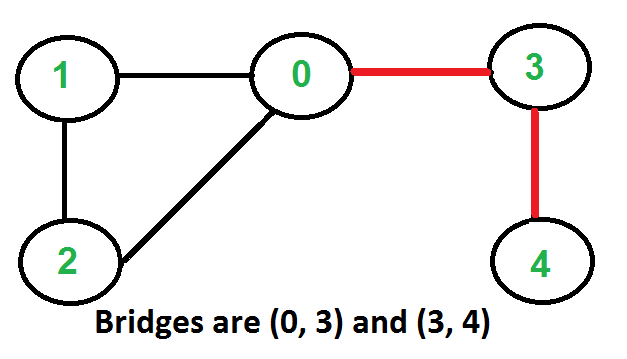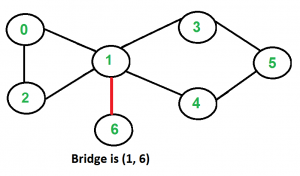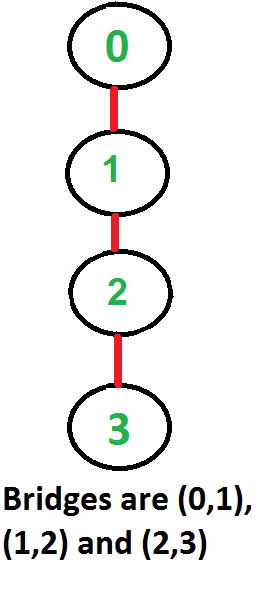GeeksforGeeks App
Open AppBrowser
Continue

# Bridges in a graph

Given an undirected Graph, The task is to find the Bridges in this Graph.

An edge in an undirected connected graph is a bridge if removing it disconnects the graph. For a disconnected undirected graph, the definition is similar, a bridge is an edge removal that increases the number of disconnected components.

Like Articulation Points, bridges represent vulnerabilities in a connected network and are useful for designing reliable networks.

Examples:

Input:Output: (0, 3) and (3, 4)

Input:Output: (1, 6)

Input:Output: (0, 1), (1, 2), and (2, 3)

Naive Approach: Below is the idea to solve the problem:

One by one remove all edges and see if the removal of an edge causes a disconnected graph.

Follow the below steps to Implement the idea:

• For every edge (u, v), do the following:
• Remove (u, v) from the graph
• See if the graph remains connected (either uses BFS or DFS)
• Add (u, v) back to the graph.

Time Complexity: O(E*(V+E)) for a graph represented by an adjacency list.
Auxiliary Space: O(V+E)

## Find Bridges in a graph using Tarjan’s Algorithm.

Before heading towards the approach understand which edge is termed as bridge. Suppose there exists a edge from u -> v, now after removal of this edge if v can’t be reached by any other edges then u -> v edge is bridge. Our approach is based on this intuition, so take time and grasp it.

ALGORITHM: –

To implement this algorithm, we need the following data structures –

• visited[ ] = to keep track of the visited vertices to implement DFS
• disc[ ] = to keep track when for the first time that particular vertex is reached
• low[ ] = to keep track of the lowest possible time by which we can reach that vertex ‘other than parent’ so that if edge from parent is removed can the particular node can be reached other than parent.

We will traverse the graph using DFS traversal but with slight modifications i.e. while traversing we will keep track of the parent node by which the particular node is reached because we will update the low[node] = min(low[all it’s adjacent node except parent]) hence we need to keep track of the parent.

While traversing adjacent nodes let ‘v’ of a particular node let ‘u’ 3 cases arise –

1. v is parent of u then,

• skip that iteration.

2. v is visited then,

• update the low of v i.e. low[u] = min( low[u] , disc[v]) this arises when a node can be visited by more than one node, but low is to keep track of the lowest possible time so we will update it.

3. v is not visited then,

• call the DFS to traverse ahead
• now update the low[u] = min( low[u], low[v] ) as we know v can’t be parent cause we have handled that case first.
• now check if ( low[v] > disc[u] ) i.e. the lowest possible to time to reach ‘v’ is greater than ‘u’ this means we can’t reach ‘v’ without ‘u’ so the edge   u -> v is a bridge.

Below is the implementation of the above approach:

## C++

 `// A C++ program to find bridges in a given undirected graph``#include``using` `namespace` `std;` `// A class that represents an undirected graph``class` `Graph``{``    ``int` `V;    ``// No. of vertices``    ``list<``int``> *adj;    ``// A dynamic array of adjacency lists``    ``void` `bridgeUtil(``int` `u, vector<``bool``>& visited, vector<``int``>& disc,``                                  ``vector<``int``>& low, ``int` `parent);``public``:``    ``Graph(``int` `V);   ``// Constructor``    ``void` `addEdge(``int` `v, ``int` `w);   ``// to add an edge to graph``    ``void` `bridge();    ``// prints all bridges``};` `Graph::Graph(``int` `V)``{``    ``this``->V = V;``    ``adj = ``new` `list<``int``>[V];``}` `void` `Graph::addEdge(``int` `v, ``int` `w)``{``    ``adj[v].push_back(w);``    ``adj[w].push_back(v);  ``// Note: the graph is undirected``}` `// A recursive function that finds and prints bridges using``// DFS traversal``// u --> The vertex to be visited next``// visited[] --> keeps track of visited vertices``// disc[] --> Stores discovery times of visited vertices``// parent[] --> Stores parent vertices in DFS tree``void` `Graph::bridgeUtil(``int` `u, vector<``bool``>& visited, vector<``int``>& disc,``                                  ``vector<``int``>& low, ``int` `parent)``{``    ``// A static variable is used for simplicity, we can``    ``// avoid use of static variable by passing a pointer.``    ``static` `int` `time` `= 0;` `    ``// Mark the current node as visited``    ``visited[u] = ``true``;` `    ``// Initialize discovery time and low value``    ``disc[u] = low[u] = ++``time``;` `    ``// Go through all vertices adjacent to this``    ``list<``int``>::iterator i;``    ``for` `(i = adj[u].begin(); i != adj[u].end(); ++i)``    ``{``        ``int` `v = *i;  ``// v is current adjacent of u``          ` `          ``// 1. If v is parent``          ``if``(v==parent)``            ``continue``;``      ` `          ``//2. If v is visited``          ``if``(visited[v]){``          ``low[u]  = min(low[u], disc[v]);``        ``}``      ` `          ``//3. If v is not visited``          ``else``{``          ``parent = u;``          ``bridgeUtil(v, visited, disc, low, parent);``  ` `          ``// update the low of u as it's quite possible``          ``// a connection exists from v's descendants to u``          ``low[u]  = min(low[u], low[v]);``          ` `          ``// if the lowest possible time to reach vertex v``          ``// is greater than discovery time of u it means``          ``// that v can be only be reached by vertex above v``          ``// so if that edge is removed v can't be reached so it's a bridge``          ``if` `(low[v] > disc[u])``              ``cout << u <<``" "` `<< v << endl;``          ` `        ``}``    ``}``}` `// DFS based function to find all bridges. It uses recursive``// function bridgeUtil()``void` `Graph::bridge()``{``    ``// Mark all the vertices as not visited disc and low as -1``    ``vector<``bool``> visited (V,``false``);``    ``vector<``int``> disc (V,-1);``      ``vector<``int``> low (V,-1);``    ` `      ``// Initially there is no parent so let it be -1``      ``int` `parent = -1;` `    ``// Call the recursive helper function to find Bridges``    ``// in DFS tree rooted with vertex 'i'``    ``for` `(``int` `i = 0; i < V; i++)``        ``if` `(visited[i] == ``false``)``            ``bridgeUtil(i, visited, disc, low, parent);``}` `// Driver program to test above function``int` `main()``{``    ``// Create graphs given in above diagrams``    ``cout << ``"\nBridges in first graph \n"``;``    ``Graph g1(5);``    ``g1.addEdge(1, 0);``    ``g1.addEdge(0, 2);``    ``g1.addEdge(2, 1);``    ``g1.addEdge(0, 3);``    ``g1.addEdge(3, 4);``    ``g1.bridge();` `    ``cout << ``"\nBridges in second graph \n"``;``    ``Graph g2(4);``    ``g2.addEdge(0, 1);``    ``g2.addEdge(1, 2);``    ``g2.addEdge(2, 3);``    ``g2.bridge();` `    ``cout << ``"\nBridges in third graph \n"``;``    ``Graph g3(7);``    ``g3.addEdge(0, 1);``    ``g3.addEdge(1, 2);``    ``g3.addEdge(2, 0);``    ``g3.addEdge(1, 3);``    ``g3.addEdge(1, 4);``    ``g3.addEdge(1, 6);``    ``g3.addEdge(3, 5);``    ``g3.addEdge(4, 5);``    ``g3.bridge();` `    ``return` `0;``}`

## Java

 `// A Java program to find bridges in a given undirected graph``import` `java.io.*;``import` `java.util.*;``import` `java.util.LinkedList;` `// This class represents a undirected graph using adjacency list``// representation``class` `Graph``{``    ``private` `int` `V;   ``// No. of vertices` `    ``// Array  of lists for Adjacency List Representation``    ``private` `LinkedList adj[];``    ``int` `time = ``0``;``    ``static` `final` `int` `NIL = -``1``;` `    ``// Constructor``    ``@SuppressWarnings``(``"unchecked"``)Graph(``int` `v)``    ``{``        ``V = v;``        ``adj = ``new` `LinkedList[v];``        ``for` `(``int` `i=``0``; i The vertex to be visited next``    ``// visited[] --> keeps track of visited vertices``    ``// disc[] --> Stores discovery times of visited vertices``    ``// parent[] --> Stores parent vertices in DFS tree``    ``void` `bridgeUtil(``int` `u, ``boolean` `visited[], ``int` `disc[],``                    ``int` `low[], ``int` `parent[])``    ``{` `        ``// Mark the current node as visited``        ``visited[u] = ``true``;` `        ``// Initialize discovery time and low value``        ``disc[u] = low[u] = ++time;` `        ``// Go through all vertices adjacent to this``        ``Iterator i = adj[u].iterator();``        ``while` `(i.hasNext())``        ``{``            ``int` `v = i.next();  ``// v is current adjacent of u` `            ``// If v is not visited yet, then make it a child``            ``// of u in DFS tree and recur for it.``            ``// If v is not visited yet, then recur for it``            ``if` `(!visited[v])``            ``{``                ``parent[v] = u;``                ``bridgeUtil(v, visited, disc, low, parent);` `                ``// Check if the subtree rooted with v has a``                ``// connection to one of the ancestors of u``                ``low[u]  = Math.min(low[u], low[v]);` `                ``// If the lowest vertex reachable from subtree``                ``// under v is below u in DFS tree, then u-v is``                ``// a bridge``                ``if` `(low[v] > disc[u])``                    ``System.out.println(u+``" "``+v);``            ``}` `            ``// Update low value of u for parent function calls.``            ``else` `if` `(v != parent[u])``                ``low[u]  = Math.min(low[u], disc[v]);``        ``}``    ``}`  `    ``// DFS based function to find all bridges. It uses recursive``    ``// function bridgeUtil()``    ``void` `bridge()``    ``{``        ``// Mark all the vertices as not visited``        ``boolean` `visited[] = ``new` `boolean``[V];``        ``int` `disc[] = ``new` `int``[V];``        ``int` `low[] = ``new` `int``[V];``        ``int` `parent[] = ``new` `int``[V];`  `        ``// Initialize parent and visited, and ap(articulation point)``        ``// arrays``        ``for` `(``int` `i = ``0``; i < V; i++)``        ``{``            ``parent[i] = NIL;``            ``visited[i] = ``false``;``        ``}` `        ``// Call the recursive helper function to find Bridges``        ``// in DFS tree rooted with vertex 'i'``        ``for` `(``int` `i = ``0``; i < V; i++)``            ``if` `(visited[i] == ``false``)``                ``bridgeUtil(i, visited, disc, low, parent);``    ``}` `    ``public` `static` `void` `main(String args[])``    ``{``        ``// Create graphs given in above diagrams``        ``System.out.println(``"Bridges in first graph "``);``        ``Graph g1 = ``new` `Graph(``5``);``        ``g1.addEdge(``1``, ``0``);``        ``g1.addEdge(``0``, ``2``);``        ``g1.addEdge(``2``, ``1``);``        ``g1.addEdge(``0``, ``3``);``        ``g1.addEdge(``3``, ``4``);``        ``g1.bridge();``        ``System.out.println();` `        ``System.out.println(``"Bridges in Second graph"``);``        ``Graph g2 = ``new` `Graph(``4``);``        ``g2.addEdge(``0``, ``1``);``        ``g2.addEdge(``1``, ``2``);``        ``g2.addEdge(``2``, ``3``);``        ``g2.bridge();``        ``System.out.println();` `        ``System.out.println(``"Bridges in Third graph "``);``        ``Graph g3 = ``new` `Graph(``7``);``        ``g3.addEdge(``0``, ``1``);``        ``g3.addEdge(``1``, ``2``);``        ``g3.addEdge(``2``, ``0``);``        ``g3.addEdge(``1``, ``3``);``        ``g3.addEdge(``1``, ``4``);``        ``g3.addEdge(``1``, ``6``);``        ``g3.addEdge(``3``, ``5``);``        ``g3.addEdge(``4``, ``5``);``        ``g3.bridge();``    ``}``}``// This code is contributed by Aakash Hasija`

## Python3

 `# Python program to find bridges in a given undirected graph``#Complexity : O(V+E)`` ` `from` `collections ``import` `defaultdict`` ` `#This class represents an undirected graph using adjacency list representation``class` `Graph:`` ` `    ``def` `__init__(``self``,vertices):``        ``self``.V``=` `vertices ``#No. of vertices``        ``self``.graph ``=` `defaultdict(``list``) ``# default dictionary to store graph``        ``self``.Time ``=` `0`` ` `    ``# function to add an edge to graph``    ``def` `addEdge(``self``,u,v):``        ``self``.graph[u].append(v)``        ``self``.graph[v].append(u)`` ` `    ``'''A recursive function that finds and prints bridges``    ``using DFS traversal``    ``u --> The vertex to be visited next``    ``visited[] --> keeps track of visited vertices``    ``disc[] --> Stores discovery times of visited vertices``    ``parent[] --> Stores parent vertices in DFS tree'''``    ``def` `bridgeUtil(``self``,u, visited, parent, low, disc):` `        ``# Mark the current node as visited and print it``        ``visited[u]``=` `True` `        ``# Initialize discovery time and low value``        ``disc[u] ``=` `self``.Time``        ``low[u] ``=` `self``.Time``        ``self``.Time ``+``=` `1` `        ``#Recur for all the vertices adjacent to this vertex``        ``for` `v ``in` `self``.graph[u]:``            ``# If v is not visited yet, then make it a child of u``            ``# in DFS tree and recur for it``            ``if` `visited[v] ``=``=` `False` `:``                ``parent[v] ``=` `u``                ``self``.bridgeUtil(v, visited, parent, low, disc)` `                ``# Check if the subtree rooted with v has a connection to``                ``# one of the ancestors of u``                ``low[u] ``=` `min``(low[u], low[v])`  `                ``''' If the lowest vertex reachable from subtree``                ``under v is below u in DFS tree, then u-v is``                ``a bridge'''``                ``if` `low[v] > disc[u]:``                    ``print` `(``"%d %d"` `%``(u,v))``    ` `                    ` `            ``elif` `v !``=` `parent[u]: ``# Update low value of u for parent function calls.``                ``low[u] ``=` `min``(low[u], disc[v])`  `    ``# DFS based function to find all bridges. It uses recursive``    ``# function bridgeUtil()``    ``def` `bridge(``self``):`` ` `        ``# Mark all the vertices as not visited and Initialize parent and visited,``        ``# and ap(articulation point) arrays``        ``visited ``=` `[``False``] ``*` `(``self``.V)``        ``disc ``=` `[``float``(``"Inf"``)] ``*` `(``self``.V)``        ``low ``=` `[``float``(``"Inf"``)] ``*` `(``self``.V)``        ``parent ``=` `[``-``1``] ``*` `(``self``.V)` `        ``# Call the recursive helper function to find bridges``        ``# in DFS tree rooted with vertex 'i'``        ``for` `i ``in` `range``(``self``.V):``            ``if` `visited[i] ``=``=` `False``:``                ``self``.bridgeUtil(i, visited, parent, low, disc)``        ` ` ` `# Create a graph given in the above diagram``g1 ``=` `Graph(``5``)``g1.addEdge(``1``, ``0``)``g1.addEdge(``0``, ``2``)``g1.addEdge(``2``, ``1``)``g1.addEdge(``0``, ``3``)``g1.addEdge(``3``, ``4``)` ` ` `print` `(``"Bridges in first graph "``)``g1.bridge()` `g2 ``=` `Graph(``4``)``g2.addEdge(``0``, ``1``)``g2.addEdge(``1``, ``2``)``g2.addEdge(``2``, ``3``)``print` `(``"\nBridges in second graph "``)``g2.bridge()` ` ` `g3 ``=` `Graph (``7``)``g3.addEdge(``0``, ``1``)``g3.addEdge(``1``, ``2``)``g3.addEdge(``2``, ``0``)``g3.addEdge(``1``, ``3``)``g3.addEdge(``1``, ``4``)``g3.addEdge(``1``, ``6``)``g3.addEdge(``3``, ``5``)``g3.addEdge(``4``, ``5``)``print` `(``"\nBridges in third graph "``)``g3.bridge()`  `#This code is contributed by Neelam Yadav`

## C#

 `// A C# program to find bridges``// in a given undirected graph``using` `System;``using` `System.Collections.Generic;` `// This class represents a undirected graph ``// using adjacency list representation``public` `class` `Graph``{``    ``private` `int` `V; ``// No. of vertices` `    ``// Array of lists for Adjacency List Representation``    ``private` `List<``int``> []adj;``    ``int` `time = 0;``    ``static` `readonly` `int` `NIL = -1;` `    ``// Constructor``    ``Graph(``int` `v)``    ``{``        ``V = v;``        ``adj = ``new` `List<``int``>[v];``        ``for` `(``int` `i = 0; i < v; ++i)``            ``adj[i] = ``new` `List<``int``>();``    ``}` `    ``// Function to add an edge into the graph``    ``void` `addEdge(``int` `v, ``int` `w)``    ``{``        ``adj[v].Add(w); ``// Add w to v's list.``        ``adj[w].Add(v); ``//Add v to w's list``    ``}` `    ``// A recursive function that finds and prints bridges``    ``// using DFS traversal``    ``// u --> The vertex to be visited next``    ``// visited[] --> keeps track of visited vertices``    ``// disc[] --> Stores discovery times of visited vertices``    ``// parent[] --> Stores parent vertices in DFS tree``    ``void` `bridgeUtil(``int` `u, ``bool` `[]visited, ``int` `[]disc,``                    ``int` `[]low, ``int` `[]parent)``    ``{` `        ``// Mark the current node as visited``        ``visited[u] = ``true``;` `        ``// Initialize discovery time and low value``        ``disc[u] = low[u] = ++time;` `        ``// Go through all vertices adjacent to this``        ``foreach``(``int` `i ``in` `adj[u])``        ``{``            ``int` `v = i; ``// v is current adjacent of u` `            ``// If v is not visited yet, then make it a child``            ``// of u in DFS tree and recur for it.``            ``// If v is not visited yet, then recur for it``            ``if` `(!visited[v])``            ``{``                ``parent[v] = u;``                ``bridgeUtil(v, visited, disc, low, parent);` `                ``// Check if the subtree rooted with v has a``                ``// connection to one of the ancestors of u``                ``low[u] = Math.Min(low[u], low[v]);` `                ``// If the lowest vertex reachable from subtree``                ``// under v is below u in DFS tree, then u-v is``                ``// a bridge``                ``if` `(low[v] > disc[u])``                    ``Console.WriteLine(u + ``" "` `+ v);``            ``}` `            ``// Update low value of u for parent function calls.``            ``else` `if` `(v != parent[u])``                ``low[u] = Math.Min(low[u], disc[v]);``        ``}``    ``}`  `    ``// DFS based function to find all bridges. It uses recursive``    ``// function bridgeUtil()``    ``void` `bridge()``    ``{``        ``// Mark all the vertices as not visited``        ``bool` `[]visited = ``new` `bool``[V];``        ``int` `[]disc = ``new` `int``[V];``        ``int` `[]low = ``new` `int``[V];``        ``int` `[]parent = ``new` `int``[V];`  `        ``// Initialize parent and visited, ``        ``// and ap(articulation point) arrays``        ``for` `(``int` `i = 0; i < V; i++)``        ``{``            ``parent[i] = NIL;``            ``visited[i] = ``false``;``        ``}` `        ``// Call the recursive helper function to find Bridges``        ``// in DFS tree rooted with vertex 'i'``        ``for` `(``int` `i = 0; i < V; i++)``            ``if` `(visited[i] == ``false``)``                ``bridgeUtil(i, visited, disc, low, parent);``    ``}` `    ``// Driver code``    ``public` `static` `void` `Main(String []args)``    ``{``        ``// Create graphs given in above diagrams``        ``Console.WriteLine(``"Bridges in first graph "``);``        ``Graph g1 = ``new` `Graph(5);``        ``g1.addEdge(1, 0);``        ``g1.addEdge(0, 2);``        ``g1.addEdge(2, 1);``        ``g1.addEdge(0, 3);``        ``g1.addEdge(3, 4);``        ``g1.bridge();``        ``Console.WriteLine();` `        ``Console.WriteLine(``"Bridges in Second graph"``);``        ``Graph g2 = ``new` `Graph(4);``        ``g2.addEdge(0, 1);``        ``g2.addEdge(1, 2);``        ``g2.addEdge(2, 3);``        ``g2.bridge();``        ``Console.WriteLine();` `        ``Console.WriteLine(``"Bridges in Third graph "``);``        ``Graph g3 = ``new` `Graph(7);``        ``g3.addEdge(0, 1);``        ``g3.addEdge(1, 2);``        ``g3.addEdge(2, 0);``        ``g3.addEdge(1, 3);``        ``g3.addEdge(1, 4);``        ``g3.addEdge(1, 6);``        ``g3.addEdge(3, 5);``        ``g3.addEdge(4, 5);``        ``g3.bridge();``    ``}``}` `// This code is contributed by Rajput-Ji`

## Javascript

 ``

Output

```Bridges in first graph
3 4
0 3

Bridges in second graph
2 3
1 2
0 1

Bridges in third graph
1 6```

Time Complexity: O(V+E),

• The above approach uses simple DFS along with Tarjan’s Algorithm.
• So time complexity is the same as DFS which is O(V+E) for adjacency list representation of the graph.

Auxiliary Space: O(V) is used for visited, disc and low arrays.

My Personal Notes arrow_drop_up